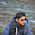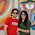## August 13, 2012

### Lazy propagation in Segment Tree

UPDATE 1: I've written a tutorial explaining basics of Segment tree along with example. You can find it at https://techienotes.info/2015/08/31/segment-tree-tutorial/

UPDATE 2: I've re-written this tutorial about lazy propagation. That updated article explains the technique in a much clear manner with a lot of comments in the code. I strongly recommend you to go through the new article. You can find it at https://techienotes.info/2015/09/01/lazy-propagation-in-segment-tree/

Question: An array of integers of $N$ elements from $1$ to $N$ is given. Two types of operations are to be done. You can add or subtract a value in the range of $p$ to $q$ where $1\le{p,q}\le{N}$ or you can ask for sum of elements between range of $p$ to $q$.

Solution: One can go for bruteforce but it is too slow. Suppose there are $T$ operations and each operation would require $O(n)$ in worst case for a loop $p$ to $q$. This approach leads to $O(T\cdot{N})$ complexity. If $T$ and $N$ both are $10^6$, this will lead to $O(10^{12})$ which isn't acceptable. However, this problem could be solved by other approaches like BIT(Binary Indexed Trees) and so on. I'm going to solve it by Segment Trees based on arrays. This array based Segment tree consumes more memory but it's easy to implement.
I assume you know at least how to build a Segment Tree. Suppose initially all the elements of array are zero and that's why we don't have to build it explicitly. We can start updating it. What I'm using to update the range is either lazy propagation or something very similar to it. Well whatever it is, here's the idea.
For a given range find that parent node close to root as much as possible which includes the given range completely. Now update this node with value$\times$range of this node. Range of this node means the range of array for which this node is responsible. After this, we set a flag on it. Idea behind flag is that this range will be updated when the query for sum will be fired. When there is query for sum of a range, we start with root and proceed downwards the tree. If flag is set on any node, value of this node will be updated and flag will be passed on to its children, i.e flag of this node will unset and will be set on its children. In this procedure of finding sum we update only those nodes which we encounter in our range.
Things become easy with example, so let's take this one.

Problem Statement: http://www.spoj.pl/problems/HORRIBLE/
Here's my code:

import java.io.BufferedReader;
import java.io.IOException;
import java.util.Arrays;
import java.io.InputStreamReader;
import java.util.StringTokenizer;

class horrible{
private static long tree[], helper[];
private static boolean color[];

public static void main (String [] args) throws IOException{
BufferedReader br = new BufferedReader (new InputStreamReader (System.in));
StringTokenizer st =null;
int t = Integer.parseInt(br.readLine());
for(;t>0;t--){
st = new StringTokenizer (br.readLine());
int N = Integer.parseInt(st.nextToken());
int C = Integer.parseInt(st.nextToken());
init(N);
for (int I=1;I<=C;I++){
st = new StringTokenizer(br.readLine());
if (st.nextToken().equals("0")){
int P = Integer.parseInt(st.nextToken());
int Q = Integer.parseInt(st.nextToken());
long V = Long.parseLong(st.nextToken());
update (1,1, N, P,Q, V);
}
else {
int P = Integer.parseInt(st.nextToken());
int Q = Integer.parseInt(st.nextToken());
long ans = sum_of_range (1,1,N, P,Q);
System.out.println(ans);
}
}
}
}

private static void init(int N){
tree = new long ;
helper = new long ;
color  = new boolean ;
Arrays.fill (tree,0);
Arrays.fill (helper, 0);
Arrays.fill (color, false);
}

private static   void update (int node, int start, int end, int left, int right, long value){
if (left > end || right <start )
return ;
if (start == end ){
tree[node]+= value;
if (color[node]==true){
tree[node] += helper[node];
helper[node]=0;
}
return;
}
if (left <=start && right >= end){
helper[2*node] += value;
helper[2*node+1] += value;
color[2*node] = color[2*node+1] = true;
tree[node] += (value *(end-start+1));
return ;
}
else if (left <= end && right >end)
tree[node ] += (value*(end-left+1));
else if (start <= right && left <start)
tree[node] += (value*(right-start+1));
else if (left >= start && right <= end)
tree[node] += (value*(right-left+1));

update (2*node, start, (start+end)/2, left, right, value);
update ((2*node)+1, ((start+end)/2)+1, end, left, right, value);
return;
}

private static long sum_of_range (int node, int start, int end, int left, int right){
if (left > end || right <start)
return 0;
if (color[node] == true){
tree[node] += (helper[node] *(end-start+1));
color[node]=false;
if (start != end){
color[2*node]=color[2*node+1] = true;
helper[2*node] += helper[node];
helper[2*node+1] += helper[node];
}
helper[node]=0;
}
if (left <= start && right >= end){
return tree[node];
}
long s1 = sum_of_range(2*node, start, (start+end)/2, left, right);
long s2 = sum_of_range(2*node+1,((start+end)/2)+1,end, left,right);
return s1+s2;
}
}



First of all, size of tree which is $262145$ isn't random figure. We build a segment tree for a given array with with $N$ leaf nodes. For a binary tree with $N$ leaf nodes its height would be $\lceil(\log_2N)\rceil$. So we need space of tree$[2^{\lceil(\log_2N)\rceil + 1}]$.Here $+1$ is because at $n^{th}$ level of binary tree number of elements is almost equal to the number of all nodes from root node to (n-1)th level in worst case so you need one extra level of height. In this case $N = 10^5$, $\lceil(\log_210^5)\rceil+1 = 18$ and $2^{18} = 262144$. Well here figure is $262145$, it's because in my practice. I prefer to allocate one more element to reduce off-by-one errors. Next, there are two other arrays helper and color. color is a boolean array to set/unset the flag on node and helper array is for storing the values in buffer until a query for sum is fired. e.g. there are five consecutive update queries and there is a node which is part of all of these queries. Suppose the five queries were to update the range by $2, 4, 1, 6, 3$. Now for every update query these values will added to helper. After these queries helper[node] will have value of $2+4+1+6+3=16$ assuming initially it was zero. Say, sixth query is for sum. Now, for e.g. if this node has range $6$ to $10$ then after sum query it will be $16\times(10-6+1) = 80$. $10-6+1$ is because this node is responsible for array element $6,7,8,9$ and $10$; a total of five elements. Do not forget to set the helper[node] to $0$ after updation. This approach is called lazy because any node won't be updated until it's needed. The values propagates from root to leaf nodes in lazy way.
Rest of the details could be easily figured out in the code itself. If something isn't clear, please leave a comment.

#### 6 comments:

1.This comment has been removed by the author.

2.This comment has been removed by the author.

3.(left >= start && right <= end) means interval of (left, right) lies between the start and end. So the value updated in that node will be equal to the range it hold which is (right - left+1) multiplied by value.

1.yeah got it later after running on paper....this part is imp if you querySum and sum or sub operation are done prev on lower ranges

Thanks :)

4.in your update() func , for below if condition(start==end)... you need to make color[node]=false if it was true before.

if (start == end ){
tree[node]+= value;
if (color[node]==true){
tree[node] += helper[node];
helper[node]=0;
}
return;
}

5.#include
#include
using namespace std;

int n;

void query1(int node,int s,int e,int b,int c,int v);
int query(int node,int s,int e,int b,int c,int off);

struct nodee{
int sum;
int offset;
}A;

int initialize(int k)
{
for(int i=0;i<=k;i++)
A[i].sum=0,A[i].offset=0;
}

int main()
{

int t,a,b,c;
cin>>t;
while(t--)
{

int n,C;

cin>>n>>C;
initialize(3*n);
//memset(A,0,n);
while(C--)
{
cin>>a>>b>>c;
if(a==0)
{
int v;
cin>>v;
query1(1,0,n,b,c,v);
}

else
cout<e)||(s>c)||(ec))
return;
else if((s>=b)&&(e<=c))
{
A[node].sum+=(v*(e-s+1));
A[node].offset+=v;
return;
}
query1(2*node,s,(s+e)/2,b,c,v);
query1(2*node+1,(s+e)/2+1,e,b,c,v);
A[node].sum=A[2*node].sum + A[2*node+1].sum + A[node].offset*(e-s+1);
}

int query(int node,int s,int e,int b,int c,int off)
{

// cout<<"hii "<< s<< " "<e)||(ec))
return 0;

else
if((s>=b)&&(e<=c))
return (A[node].sum+off*(e-s+1));

off+=A[node].offset;
return (query(2*node,s,(s+e)/2,b,c,off)+query(2*node+1,(s+e)/2+1,e,b,c,off));
}